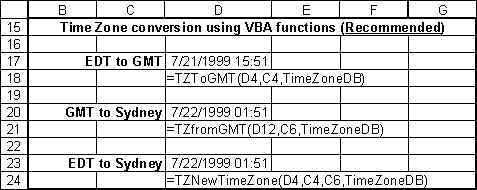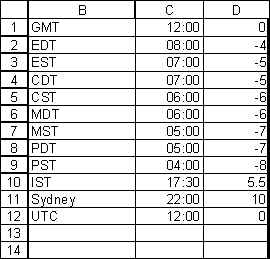You are on the Home/Excel/Time Zone Conversion pageShare Your

 Examples of converting from one time zone to another How do I set up the necessary files? How do I customize the conversion database? The spreadsheet formulas in detail The VBA functions in detail Future Plans Download the TimeZoneConvert.zip file

## TimeZone conversion examples

Before describing how the conversion is accomplished here are two examples.  The first uses spreadsheet formulas only.  The advantage of this method is that no VBA code is required.  The disadvantage is that it is cumbersome to add the conversion formulas in multiple cells.  The second method -- and the preferred method -- uses a VBA add-in to achieve its goals.

The example below converts the time in cell \$D\$4, which as cell \$C\$4 indicates is Eastern Daylight Time (EDT).  The corresponding time in Sydney (cell \$C\$6) is shown in cell \$D\$6.Cells \$D\$5 and \$E\$5 contain intermediate formulas (described in xx).

This method is much easier to use.  The add-in includes the three functions described in the example below.  The first, TZToGMT converts any time to GMT (also known as UTC).  The second function, TZFromGMT converts any time from GMT to the local time in a specified time zone.  The third function TZNewTimeZone converts the local time in one zone to the local time in another zone.

Cell \$D\$17 contains the function =TZToGMT (D4,C4,TimeZoneDB). It converts the time in  \$D\$4 (which, as specified in cell  \$C\$4, happens to be EDT time) to GMT.

Similarly, the function =TZFromGMT (D12,C6,TimeZoneDB) in cell \$D\$20 contains the result of converting GMT time to the local time in Syndey, Australia.

Finally, consider the function =TZNewTimeZone (D4,C4,C6, TimeZoneDB) in cell \$D\$23.  It converts the time in cell \$D\$4, which happens to be EDT time (specified in cell \$C\$4) to the time in Syndey, Australia (specified in cell \$C\$6).## Setting up time zone conversions in Excel

Option 1 (recommended):   Download the file TimeZoneConvert.zip.  After unzipping the file, there will be two files on your disk -- TimeZoneConvert.xla, and TimeZoneExamples.xls.  Install the TimeZoneConvert.xla as an add-in.  This will add three user-defined functions that you can use.  The functions are TZToGMT, TZFromGMT, and TZNewTimeZone (see the examples section on how to use them).

The TimeZoneExamples.xls, in addition to containing examples also contains the database for conversion.  The file must be open for you to use the conversion functions! [In the next version of the functions, the database will be part of the add-in itself.]

Option 2:  Download the file TimeZoneConvert.zip.  After unzipping the file, there will be two files on your disk -- TimeZoneConvert.xla, and TimeZoneExamples.xls.  Open the TimeZoneExamples.xls file and copy the spreadsheet formulas as you need them.

Again, the TimeZoneExamples.xls, in addition to containing examples also contains the database for conversion.  The file must be open for you to use the conversion functions! [In the next version of the functions, the database will be part of the add-in itself.]

Option 3:  You can copy the code from the Web pages and follow the directions as described in the sections, "The TimeZone VBA functions" or "The TimeZone spreadsheet functions"

Note that both options 2 and 3 will require that you adjust the formulas to your spreadsheet.

## The TimeZone database

The database used for converting from one time zone to another is actually quite straightforward.  It is in the file TimeZoneExamples.xls (which is why that file must be open before any of the functions in TimeZoneConvert.xla can be used).  For each time zone the database contains three pieces of information.

• The first (in column B in the figure below) is how the time zone is identified.  For example, Eastern Daylight Time is called EDT, while Greenwhich Mean Time is GMT.  Note that UTC is another way to refer to GMT.
• The second piece of information (in column C) is the local time (using a 24 hour clock) corresponding to midday GMT.  For example, when it is 12:00 midday in GMT, it is 08:00 EDT (which is 8:00 a.m.).  Similarly, midday GMT is 22:00 in Sydney, Australia (which is 10:00 p.m.).
• The third piece of information is a formula that calculates the time difference between midday GMT and the corresponding local time.#### To add a new time zone

In the first empty cell in column B enter how you want to identify the new time zone.  In the next cell (in column C) enter the local. time (using a 24 hour clock) corresponding to midday GMT.  Finally, copy the formula from the cell in column D of the the prior row to the cell in column D of the current row.   You are now ready to use the new time zone in your calculations.

#### How the 24 hour clock works

Times between midnight and midday are represented in the 'normal' fashion, i.e., the time goes from 00:00 (midnight) through 03:00 (3 a.m.) to 08:00 (8 a.m.) to 12:00 (midday).

To convert time from a 12 hour clock to a 24 hour clock, add 12 to the time in p.m.   So, the time goes from 12:00 (midday) to 13:00 (1 p.m.) to 18:00 (6 p.m.) to 21:00 (9 p.m.) to 23:00 (11 p.m.) to 00:00 (midnight).

## The TimeZone VBA functions

TZToGMT converts from local time to GMT by calling the following function:

Function ToGMT(aTime As Date, fromCode As String, TimeZoneDB As Range)
'aTime contains a Date/Time that needs to the converted to GMT _
fromCode is a time zone id (such as EDT) associated with aTime _
and TimeZoneDB is a 2-D range with the time difference between GMT _
and various time zones.  The layout is: _
col. 1         col. 2               col. 3 _
GMT             12:00 (midday)       local time - GMT _
<local id>     time corr. to        local time - GMT _
midday GMT
Dim rslt, temp1
rslt = -Application.WorksheetFunction.VLookup(fromCode, TimeZoneDB, 3, False)
On Error GoTo 0
temp1 = TimeValue(Fix(Abs(rslt)) & ":" & (Abs(rslt) - Fix(Abs(rslt))) * 60 & ":0")
ToGMT = aTime + Sgn(rslt) * temp1
Exit Function
BadTimeZoneCode: ToGMT = "Unknown time zone:" & fromCode
End Function    'ToGMT

TZFromGMT converts from GMT to a local time by calling the following function:

Function FromGMT(aTime As Date, toCode As String, TimeZoneDB As Range)
'see toGMT for an explanation of parameters
Dim rslt, temp1
rslt = Application.WorksheetFunction.VLookup(toCode, TimeZoneDB, 3, False)
On Error GoTo 0
temp1 = TimeValue(Fix(Abs(rslt)) & ":" & (Abs(rslt) - Fix(Abs(rslt))) * 60 & ":0")
FromGMT = aTime + Sgn(rslt) * temp1
Exit Function
BadTimeZoneCode: FromGMT = "Unknown time zone:" & toCode
End Function    'FromGMT

TZNewTimeZone converts from one local time to another by first calling the TZToGMT function above and then using that result to call the TZFromGMT function.

The spreadsheet fomula for converting the time from one time zone to another works very similarly to how the VBA function TZNewTimeZone works.   However, given the various checks and corrections needed, the calculation is split into three cells (where the first two contain intermediate results).

An example calculation is shown below:The first intermediate formula (in cell \$D\$5) calculates the time difference (in hours) between the timezones (given in \$C\$4 -- EDT -- and \$C\$6 -- Sydney).  The formula is:

=-VLOOKUP(C4,TimeZoneDB,3,FALSE) +VLOOKUP(C6,TimeZoneDB,3,FALSE)

The second intermediate formula (in cell \$E\$5) converts the time difference into a form that Excel interprets as a time value

=TIME(TRUNC(ABS(D5)),(ABS(D5) -TRUNC(ABS(D5)))*60,0)

The final result is assembled in cell \$D\$6:

=IF(ISERROR(D5),"Unknown timezone", IF(D5<0,IF(D4<E5,D4-E5+2*TIME(12,0,0),D4-E5),D4+E5))

## Future Plans

The immediate plan is to integrate the database (which is currently in TimeZoneExamples.xls) into the TimeZoneConvert.xla add-in.  At that time, a new macro, to facilitate maintenance of the database,  will be included.